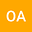LOCAL FRACTIONAL REDUCED DIFFERENTIAL TRANSFORM METHOD FOR SOLVING FRACTAL HEAT CONDUCTION EQUATIONS
•• Omer Acan
Omer Acan
Siirt Universitesi

Corresponding Author:[email protected]

Author Profile## Abstract

In this study, time-space fractional heat conduction equation (HCEs), which plays an important role in thermal science, is considered on Cantor set. The analytical solution of this equation is obtained by using local fractional reduced differentiable transform method in fractal spaces. After giving preliminaries, some definitions and fundamental properties belong to this procedure are given. Then to make it easy to understand, this method is applied to homogeneous and non-homogeneous time-space fractional HCEs and analytic solutions are obtained. After that, physical behaviours of the solutions on fractal spaces are illustrated in 3D graphics. This shows the efficiency and reliability of the method.